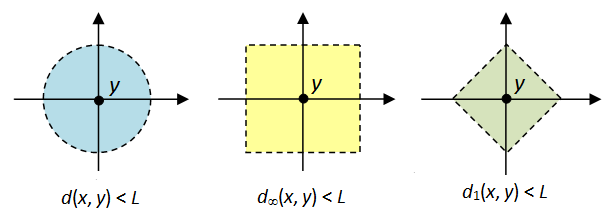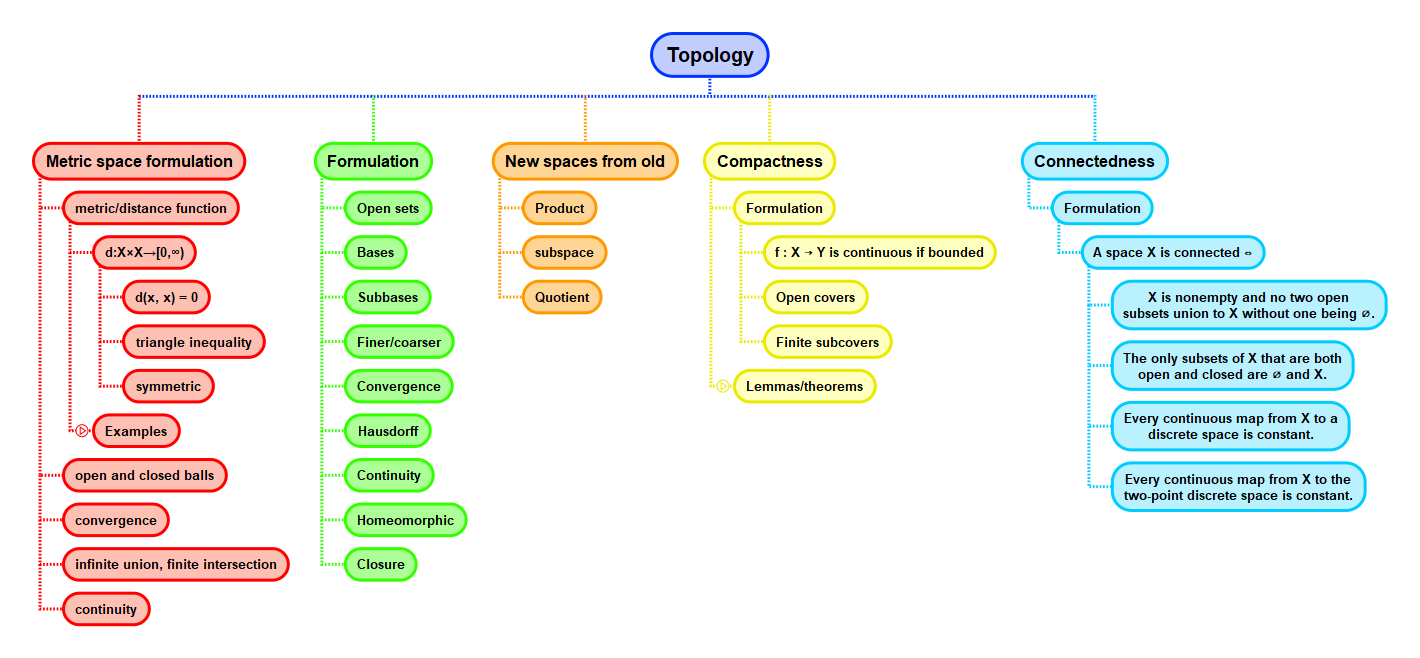Show Question
Math and science::Topology

# Metric space. ε-balls

Let $$X$$ be a metric space, let $$x \in X$$ and let $$\varepsilon > 0$$ be a real. The open ε-ball around $$x$$ (or in more detail, the open ball around $$x$$ of radius $$\varepsilon$$) is the subset of $$X$$ given by

$$B(x, \varepsilon) = \{y \in X : d(x, y) < \varepsilon \}$$

Similarly, the closed ε-ball around $$x$$ is

$$\bar{B}(x, \varepsilon) = \{y \in X : d(x, y) \le \varepsilon \}$$

The definition of open sets in metric spaces is formulated in terms of open ε-balls.

### Example

Metrics such as $$d_1$$, $$d_2$$ and $$d_\infty$$ for both $$\mathbb{R}^n$$ and continuous function spaces $$C[a, b]$$, and other metrics such as Hamming distance, each induce ε-balls within the space they are applied in. It is interesting to consider what the ε-ball in each metric space is.## Context